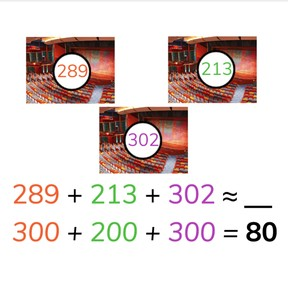Estimate the sum to 1,000

# Estimate the sum to 1,000

No account needed.8,000 schools use Gynzy92,000 teachers use Gynzy1,600,000 students use Gynzy

## General

Students learn to estimate the sum with totals to 1,000 by rounding tens, hundreds and hundred tens.

2.NBT.B.7

## Relevance

Discuss with students that it is important to be able to estimate totals because it makes it easier to know about how many there are. This can be used if you want to quickly know about how many students are in three classes.

## Introduction

Start by having students set three numbers to 1,000 on the number line and to come up with their closest tens number. Then practice rounding different numbers to either 400 or 500 Ask students to determine if a number is closer to a hundreds (300) or a hundred ten (320). Ask students how they know how to round a number.

## Development

Explain to students that when you estimate the total you round your addends to a round number and then add them together. The addition problem 199+402 becomes 200+400. Explain, using a different example that you sometimes can round to a tens and sometimes to a hundred. With hundreds it is easier, but rounding with tens gets you closer to the actual answer. Discuss with students that when you want to be fast you can estimate more globally and when you want to be more precise you can round in tens to give a more accurate estimation. Show different numbers that you can easily round to a hundred and easily round to a ten and show how the totals vary in different addition problems. Next give an example in which they must estimate with money- in a real world context. Ask if they have enough money for the bag and shoes. Practice being as precise as possible with your estimation.

To check that students can estimate a sum up to 1,000 you can ask the following questions:
- Why is it useful to be able to estimate?
- What do you do when you want to estimate a total?
- Why is it sometimes better to round to a ten, or better to round to a hundred when estimating a total?

## Guided practice

Students first practice with some addition problems in which they round to the nearest hundred. Then they are given problems in which they should round to tens, and finally they must estimate with money amounts in a story problem.

## Closing

Discuss with students that it is useful to be able to estimate sums so you can quickly determine about how much you have. Discuss why you sometimes round to a tens, and sometimes to a hundred. The more precisely you round, the closer your estimation will be. Discuss a few statements with the students and ask the students if they agree if they should estimate or not. Practice a few estimation addition problems and check that students are able to estimate the sum with totals to 1,000.

## Teaching tips

Students who have trouble with estimating sums can first practice rounding numbers to 1,000. Once they are more comfortable rounding to tens and hundreds they can solve these problems easier. If a student takes a long time to solve these problems, make sure that they are not solving the exact addition problem and rounding the total, but that they are rounding the addends before adding.

### The online teaching platform for interactive whiteboards and displays in schools

• Save time building lessons

• Manage the classroom more efficiently

• Increase student engagement